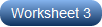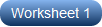1. Worksheets>
2. Math>
3. Pre-Algebra>
5. Rationalizing the Denominators

# Rationalizing the Denominators Worksheets

Fractions cannot have irrational radicals (or surds) in the denominator. We use a technique called rationalization to eliminate them. Keep students informed of the steps involved in this technique with these pdf worksheets offering three different levels of practice. Level 1 introduces radical expressions that consist of a single term in the denominator, level 2 presents expressions with single and two terms in the denominator, and level 3 delivers radicals involving single and two terms in the numerator of the fractions. Take advantage of our free worksheet and commence practice!

Rationalize the Denominators - Level 1

Work your way through these pdf worksheets to hone your skills in rationalizing the denominators. Multiply the numerator and denominator by the given radical to have a rational number in the denominator, and further simplify the expression.Rationalize the Denominators - Level 2

Be one step ahead of your peers with these printable worksheets. In order to rationalize the denominator, multiply the conjugate of the denominator to both the numerator and denominator and simplify the expressions using the FOIL method.Rationalize the Denominators - Level 3

Reiterate the concept of rationalizing denominators with these high school worksheets. The questions in this level are a little more challenging than those in the first two. Rationalize the denominators carefully, and check your responses.Related Worksheets